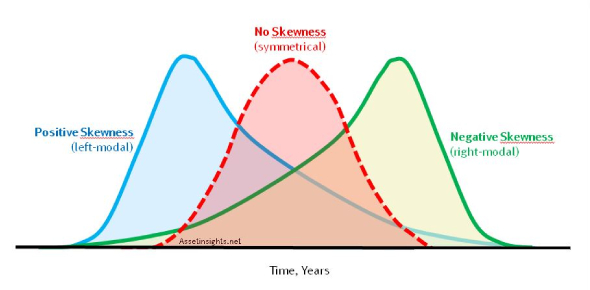# Probability Distribution Quiz Exam!

10 Questions | Total Attempts: 89Settings.

• 1.
The probability distribution of a discrete random variable.
• A.

Probability mass function (pmf)

• B.

Probability density function (pdf)

• C.

Cumulative distribution function (pmf)

• 2.
The probability distribution of a continuous variable.
• A.

Probability mas function (pmf)

• B.

Probability density function (pdf)

• C.

Cumulative distribution function (cdf)

• 3.
The probability that the variable takes a value less than or equal to x.
• A.

Probability mass function (pmf)

• B.

Probability density function (pdf)

• C.

Cumulative distribution function (cdf)

• 4.
The values of the discrete random variable X where f(x) > 0
• A.

Support S

• B.

Expected value

• C.

Mass points

• 5.
The set of values that that the random variable can take__________
• A.

Expected value

• B.

Mass points

• C.

Support S

• 6.
The weighted average of the elements of a probability distribution.
• A.

Expected value

• B.

Support S

• C.

Mass points

• 7.
A random variable X has a probability distribution as follows x 0 1 2 3 4 P(x) 0.06 0.23 k 0.35 0.05 Find P(2)
• A.

0.30

• B.

0.31

• C.

0.23

• D.

0.13

• 8.
Using table in #7 What is P(x>2)
• A.

0.35

• B.

0.40

• C.

0.71

• D.

0.75

• 9.
The probability distribution of a discrete random variable is shown in the table below x 1 2 3 4 5 P(x) 2/25 6/25 2/5 4/25 3/5d Find d
• A.

1/5

• B.

5

• C.

2

• D.

3/25

• 10.
Given the histogram above, What is  P (x < 3)?
• A.

0.2500

• B.

0.6875

• C.

0.7125

• D.

Option 4

Related TopicsBack to top